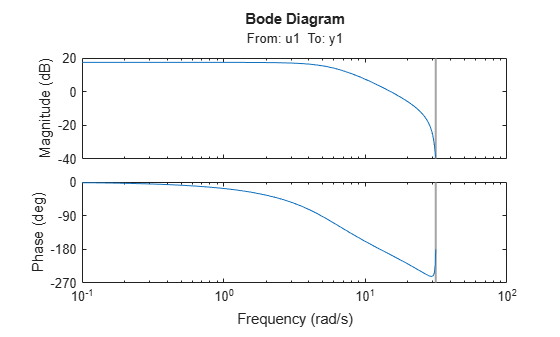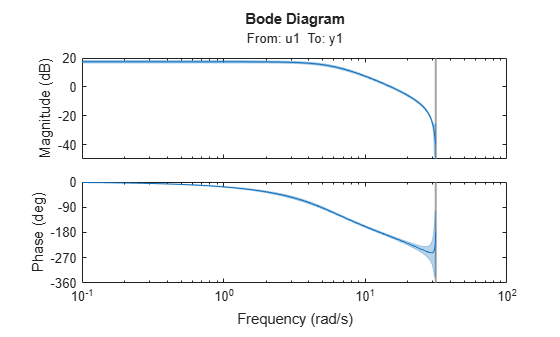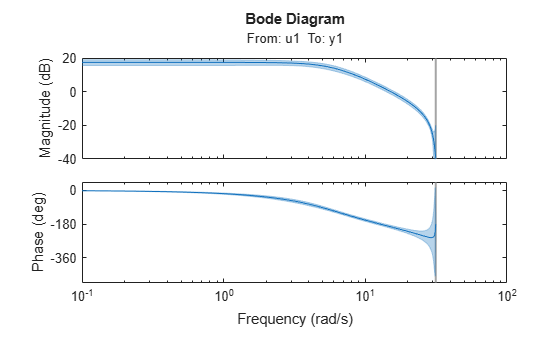showConfidence

Display confidence regions on response plots for identified models

Syntax

showConfidence(plot_handle)
showConfidence(plot_handle,sd)

Description

showConfidence(plot_handle) displays the confidence region on the response plot, with handle plot_handle, for an identified model.

showConfidence(plot_handle,sd) displays the confidence region for sd standard deviations.

Input Arguments

 plot_handle Response plot handle. plot_handle is the handle for the response plot of an identified model on which the confidence region is displayed. It is obtained as an output of one of the following plot commands: bodeplot, stepplot, impulseplot, nyquistplot, or iopzplot. sd Standard deviation of the confidence region. A common choice is 3 standard deviations, which gives 99.7% significance. Default: getoptions(plot_handle,'ConfidenceRegionNumberSD')

Examples

collapse all

Show the confidence bounds on the bode plot of an identified ARX model.

Obtain identified model and plot its bode response.

sys = arx(z1, [2 2 1]);
h = bodeplot(sys);z1 is an iddata object that contains time domain system response data. sys is an idpoly model containing the identified polynomial model. h is the plot handle for the bode response plot of sys.

Show the confidence bounds for sys.

showConfidence(h);This plot depicts the confidence region for 1 standard deviation.

Show the confidence bounds on the bode plot of an identified ARX model.

Obtain identified model and plot its bode response.

sys = arx(z1, [2 2 1]);
h = bodeplot(sys);z1 is an iddata object that contains time domain system response data. sys is an idpoly model containing the identified polynomial model. h is the plot handle for the bode response plot of sys.

Show the confidence bounds for sys using 2 standard deviations.

sd = 2;
showConfidence(h,sd);sd specifies the number of standard deviations for the confidence region displayed on the plot.

Alternatives

You can interactively turn on the confidence region display on a response plot. Right-click the response plot, and select Characteristics > Confidence Region.